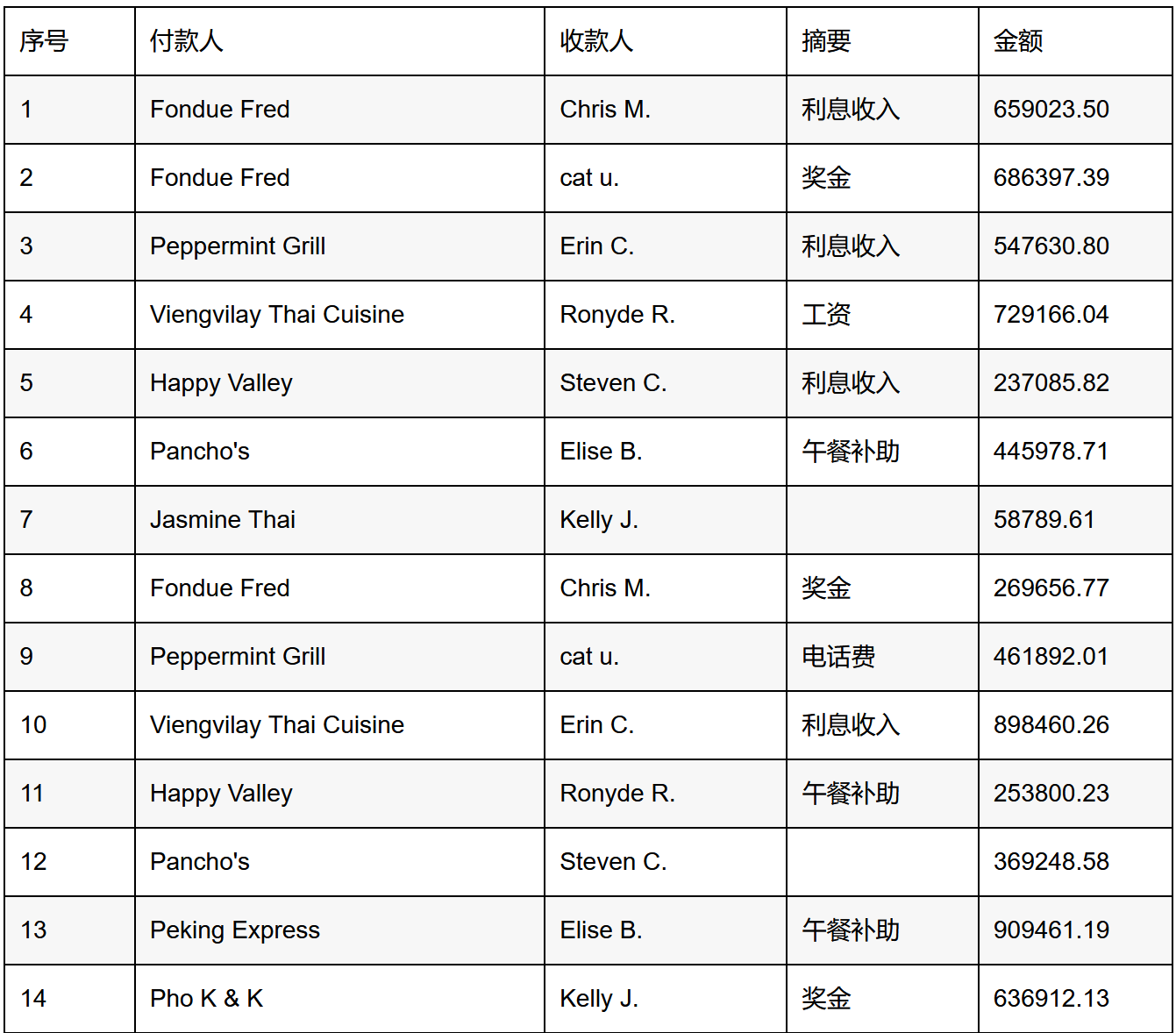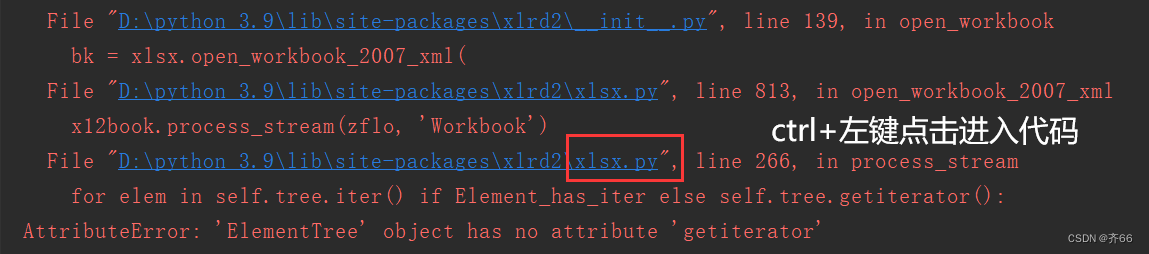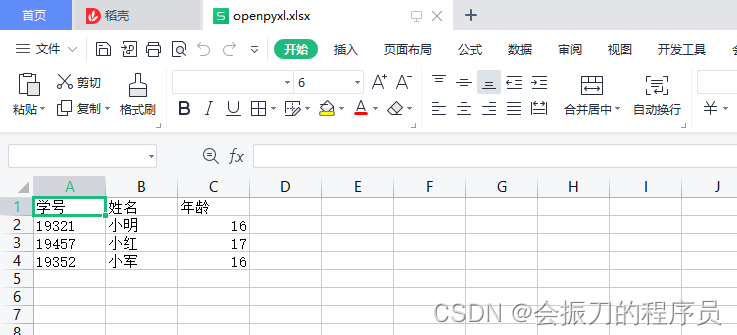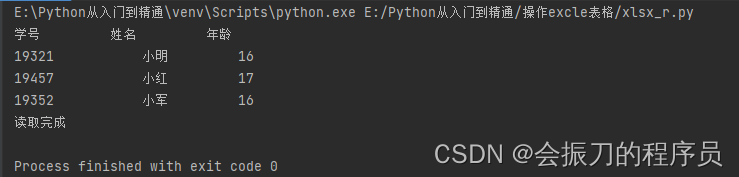# python读写excel文件

+关注继续查看

Python操作excel的三个工具包如下，注意，只能操作.xls，不能操作.xlsx。

• xlrd: 对excel进行读相关操作

• xlwt: 对excel进行写相关操作

• xlutils: 对excel读写操作的整合

• sudo pip install xlrdsudo

• pip install xlwtsudo

• pip install xlutils

xlrd是用来从一个excel文件中读取内容的模块

 导入 import xlrd 打开excel data = xlrd.open_workbook('demo.xls') #注意这里的workbook首字母是小写 查看文件中包含sheet的名称 data.sheet_names() 得到第一个工作表，或者通过索引顺序 或 工作表名称 table = data.sheets() table = data.sheet_by_index(0) table = data.sheet_by_name(u'Sheet1') 获取行数和列数 nrows = table.nrows ncols = table.ncols 获取整行和整列的值（数组） table.row_values(i) table.col_values(i) 循环行,得到索引的列表 for rownum in range(table.nrows): print table.row_values(rownum) 单元格 cell_A1 = table.cell(0,0).value cell_C4 = table.cell(2,3).value 分别使用行列索引 cell_A1 = table.row(0).value cell_A2 = table.col(1).value 简单的写入 row = 0 col = 0 ctype = 1 # 类型 0 empty,1 string, 2 number, 3 date, 4 boolean, 5 error value = 'lixiaoluo' xf = 0 # 扩展的格式化 (默认是0) table.put_cell(row, col, ctype, value, xf) table.cell(0,0) # 文本:u'xxxx' table.cell(0,0).value # 'yyyy'

xlwt只能创建一个全新的excel文件,然后对这个文件进行写入内容以及保存。但是大多数情况下我们希望的是读入一个excel文件，然后进行修改或追加，这个时候就需要xlutils了。

 导入xlwt import xlwt 新建一个excel文件 file = xlwt.Workbook() #注意这里的Workbook首字母是大写，无语吧 新建一个sheet table = file.add_sheet('sheet name') 写入数据table.write(行,列,value) table.write(0,0,'test') 如果对一个单元格重复操作，会引发 returns error:# Exception: Attempt to overwrite cell:# sheetname=u'sheet 1' rowx=0 colx=0 所以在打开时加cell_overwrite_ok=True解决 table = file.add_sheet('sheet name',cell_overwrite_ok=True) 保存文件 file.save('demo.xls') 另外，使用style style = xlwt.XFStyle() #初始化样式 font = xlwt.Font() #为样式创建字体 font.name = 'Times New Roman' font.bold = True style.font = font #为样式设置字体 table.write(0, 0, 'some bold Times text', style) # 使用样式

 # -*- coding: cp936 -*- import xlwt; import xlrd; #import xlutils; from xlutils.copy import copy; import xlrd #用xlrd提供的方法读取一个已经存在的excel文件,使用"formatting_info=True"保持源文件格式不变 rexcel = xlrd.open_workbook("file.xls",formatting_info=True) #用wlrd提供的方法获得现在已有的行数 rows = rexcel.sheets().nrows #cols = rexcel.sheets().ncols #用xlutils提供的copy方法将xlrd的对象转化为xlwt的对象 excel = copy(rexcel) #用xlwt对象的方法获得要操作的excel中的表名（sheet），0表示第一张表，1表示第二张表。 table = excel.get_sheet(0) values = ['1','2','3'] row = rows print row for value in values: # xlwt对象的写方法，参数分别是行、列、值     table.write(row,0,value)     table.write(row,1,"haha")     table.write(row,2,"lala")     row+=1 # xlwt对象的保存方法，这时便覆盖掉了原来的excel   excel.save("file.xls")Python 帮同事用pandas快速筛选Excel文件
Python 帮同事用pandas快速筛选Excel文件
15 0Python Excel表格列数的字母表达与数字的相互转换
Python Excel表格列数的字母表达与数字的相互转换
12 0python 用openpyxl库修改excel表格数据（实例）
python 用openpyxl库修改excel表格数据（实例）
19 0Python 用openpyxl库从excel表格中获取内容（实例）
Python 用openpyxl库从excel表格中获取内容（实例）
13 0python合并多个excel

31 0【Python从入门到实践5.0】将数据写入Excel表格
【Python从入门到实践5.0】将数据写入Excel表格
23 0【Python从入门到实践5.1】读取Excel表格数据
【Python从入门到实践5.1】读取Excel表格数据
21 0

Python基础快速入门实战教程377988